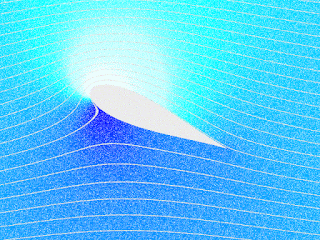## Friday, 24 January 2020

### The energy-momentum tensor in SRFluid flow by Thierry Dugnolle
Many moons ago I had skipped the end of section 9 and all of section 10 in chapter 1. It is time to return to them and flat space time starting with the end of section 9 it looks at the energy-momentum tensor for a perfect fluid. I had written at the top of the page "? don't follow much of this from 1.116". Now I do. It shows how to get from that tensor to two well known classical (non-relativistic) equations in the non-relativistic limit: a continuity equation and the Euler equation from fluid dynamics. This gives us faith that the tensor is correct - even though I had not heard of either of these equations.

Along the way we find some tricks with four-velocity $U^\mu={dx^\mu} / {d\tau}$, meet the projection tensor $P_{\ \ \ \ \nu}^\sigma$ which projects a vector orthogonal to another do some dimensional analysis and find out what energy density really is (I missed this in the book, or Carroll forgot to mention it). I had to consult the great Physics Forums on that and got liked for a facetious comment about measuring it in £sd 😀- I'm sure that oil companies must do it: kerosene is more valuable per kg than bunker fuel.

All the details here Commentary 1.9 Energy Momentum Tensor.pdf (6 pages)